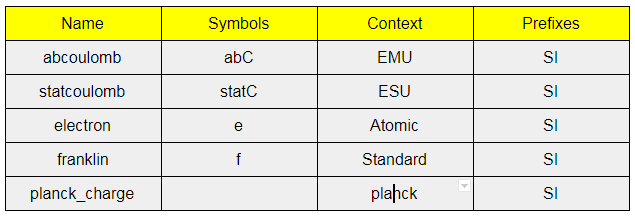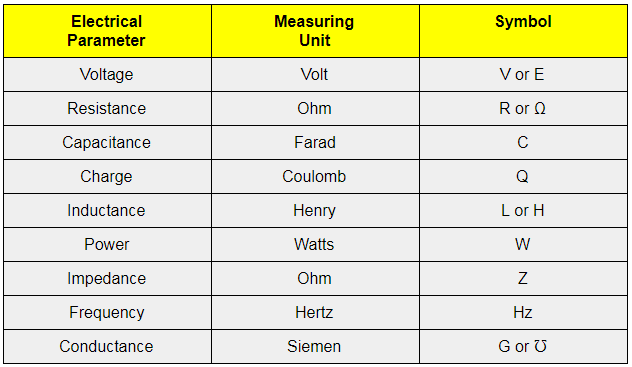# Unit of Electric Charge

There are many properties of electricity which are all expressed using different units of measurement. However, we will discuss the electric charge which is one of the electrical properties, and understand what unit is used to measure it. First, let us look at the definition of electric charge. It is defined as a physical property of matter that causes it to experience a force when placed in an electromagnetic field. Electric charge is carried by subatomic particles and there are usually positive and negative electric charges present.

## SI Unit of Electric Charge

The SI unit of electric charge is the coulomb which is a derived SI unit and is represented by the symbol C. A coulomb is defined as the amount of charge that passes via electrical conductor carrying one ampere per second.

Additionally, the cgs unit of electric charge is expressed as a combination of three fundamental quantities like mass, length, and time.

### Other Electric Charge Units

Some of the other units of electric charge are listed in the following table.#### Popular Electrical Units

Some of the units that are used to measure electrical properties are given below.Related articles:

Test your knowledge on Unit Of Electric Charge